2017-12-15

Package irtoys is a collection of functions related to Item Response Theory (IRT), (Hambleton and Swaminathan 1985), (Embretson and Reise 2000). With a bit of knowledge of R, these can be used in practice and combined into larger programs. The diminutive “toys” in the title was supposed to evoke LEGO stones rather than dolls and teddy bears.

As of the time irtoys was published, IRT models could be fit with various programs employing diverse and often arcane syntax. Mastering such a syntax took time, and learning several in order to compare results across programs could be a challenge. One of the purposes of irtoys was to offer a simple and unified R interface to some of these programs, just enough to be able to start immediately. irtoys supports a common and basic subset of the features of three estimation programs: R package ltm (Rizopoulos 2006), Brad Hanson’s freely available program, ICL (Hanson 2002), and the commercially available program, BILOG-MG (Zimowski et al. 1996). Basically, the estimation of the 1PL, 2PL, and 3PL models is supported for a single test form of dichotomously scored items. Users with more complex problems could use the syntax generated by irtoys as a starting point in learning one of these programs in depth. Alternatively, they could use some of the R packages that have emerged in the meanwhile, such as mirt (Chalmers 2012), TAM (Kiefer, Robitzsch, and Wu 2016), eRm (Mair and Hatzinger 2007), and others.

The functions in irtoys cover a wide range of tasks, including but not limited to ability estimation (maximum likelihood, modal likelihood, EAP, weighted likelihood) or IRT scaling – thanks to Tamaki Hattori, it is probably the only package to offer the Haebara’s method in its entirety, as described in (Haebara 1980). But irtoys is particularly strong in item fit. While Bilog-style chi-square statistics are supported in an extremely flexible way, R’s strength in graphics and visualisation has enabled the creation of various plots, and the list is still being extended. irtoys was arguably the first package to offer non-parametric trace lines similar to those in Ramsay’s TestGraf program (Ramsay 2000), nowadays completely emulated by package KernSmoothIRT1 (Mazza, Punzo, and McGuire 2014).

Version 0.1.0 of irtoys adds several new features:

• several new graphical tools to evaluate item fit
• the interaction model (Haberman 2007), an important link between Classical Test Theory (CTT) and IRT
• alternative links for ICL (in the help screen for irtoys-package)
• this vignette

Because of the many functions included, this vignette will concentrate on graphical tools for assessing item fit, with special emphasis on new inclusions.

library(irtoys)

The package includes a small but real data set of 18 items (multiple-choice, 4 options scored as true or false) and 472 persons. The actual responses are provided as a data frame, Unscored, and the 0/1 scores as Scored. Because not everybody has BILOG-MG, item parameters estimates for the 1PL, 2PL and 3PL models are provided as data sets b1, b2, and b3.

The original plot method for item response functions (IRF) makes it easy to compare the trace lines under the 1PL, 2PL, and 3PL models for an arbitrary item. For some items the lines are close, while for others they can be quite different:

plot(irf(b1, items=3), main="IRF for item 3", co=2)
plot(irf(b2, items=3), co=3, add=TRUE)
plot(irf(b3, items=3), co=4, add=TRUE)

plot(irf(b1, items=13), main="IRF for item 13", co=2)
plot(irf(b2, items=13), co=3, add=TRUE)
plot(irf(b3, items=13), co=4, add=TRUE)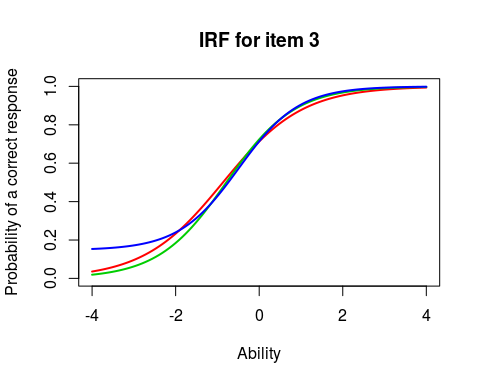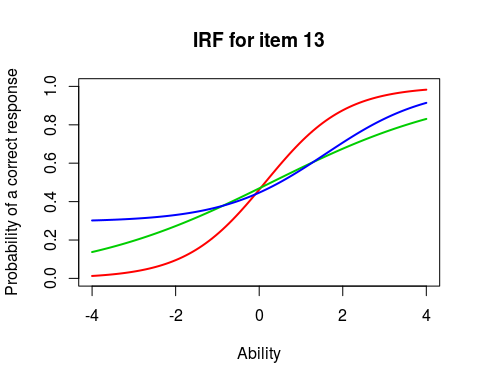The 1PL is shown in red, the 2PL in green, and the 3PL in blue.

A new function, irfPlot, tries to show the uncertainty about the lines. The delta method is used to compute the standard error of the item response function from the variance-covariance matrix of the item parameter estimates. Because ICL does not produce the variance-covariance matrix, irfPlot will not display the confidence envelopes when ICL is used to estimate the model. Another thing to note is that variance-covariance matrices produced with Bilog and ltm may differ to a larger extent than the parameter estimates themselves.

x = qrs(Scored)
irfPlot(Scored, b1, x=x, item=3, main="1PL")
irfPlot(Scored, b2, x=x, item=3, main="2PL")
irfPlot(Scored, b3, x=x, item=3, main="3PL")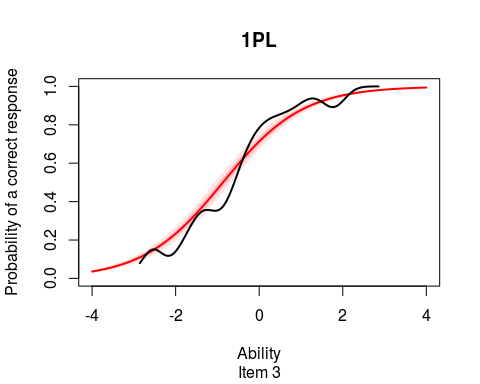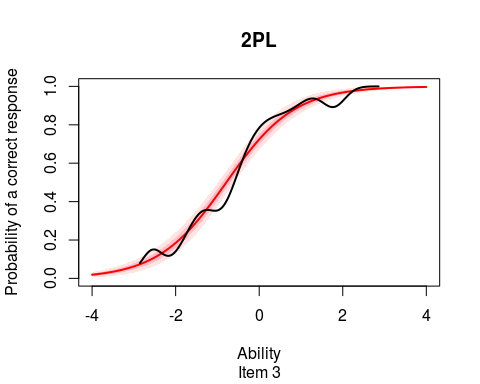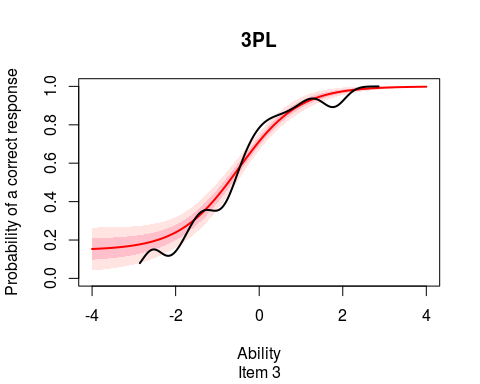The estimated trace lines are shown in red, and the 95% and 67% error bounds are in light and darker pink, accordingly. The wiggly black line is an attempt to represent the data and compare it to the model, and will be explained in a short while.

Two things are worth noticing:

First, as the model gets more complicated, the uncertainty tends to increase even though the trace lines themselves are not that different. The item is not very difficult (its difficulty is -0.49042 according to the the 3PL model), so there is little incentive to guess. The non-parametric line seems to confirm this. There is little data to estimate the asymptote, and a usual practice is to adopt a prior with the mean of $$1/k$$ where $$k$$ is the number of response alternatives. With almost no observed data in that ability range, the prior will dominate, and we end up with more bias, as shown by the discrepancy between the estimated trace line and the non-parametric curve, and more variance, as shown by the inflated confidence envelope. In statistics, we are more accustomed to a trade-off between bias and variance.

Second, while plotting a trace line is easy and adding the confidence intervals only a marginal extra effort, adding the data to the plot is quite challenging. In contrast to an ordinary regression, the model is not on the same scale as the data, but refers to an unobservable latent variable. On the plot above, the data is represented as a non-parametric regression of the probability of getting the item right on some measure of ability. The regression can be computed with package sm (Bowman and Azzalini 2014) or via the Bayes theorem, using only the density estimation routines in base R: the user has the choice. The choice of ability measure is also open: $$x$$ can be any ability estimate (MLE, BME, WLE, EAP, plausible value) based on any model (1PL, 2PL, 3PL). To avoid the suspicion of circular reasoning – use estimates under a model to assess the model’s quality? – the example above prefers function qrs, which produces a rough measure of ability due to J. Ramsay:

qrs
## function (resp)
## {
##     raw.scores = apply(resp, 1, sum, na.rm = TRUE)
##     ranks = rank(raw.scores, ties.method = "random")
##     return(as.matrix(qnorm(ranks/(length(ranks) + 1))))
## }
## <environment: namespace:irtoys>

What happens here is that the sum scores are ranked, breaking ties at random, and the ranks are transformed to Normal deviates. The result correlates quite highly with the usual estimates of ability, for example, with the WLE {@Warm] under the 2PL:

ramsay = qrs(Scored)
warm = wle(Scored, b2)[,1]
cor(ramsay, warm)
##           [,1]
## [1,] 0.9724841

The correlation is in fact quite competitive with the one between WLE under the 1PL and 2PL models, say:

cor (warm, wle(Scored, b1)[,1])
##  0.9817064

Another objection might be that the non-parametric regression is not error-free by itself. irtoys has yet another function, npp, that shows confidence bounds by courtesy of package sm (Bowman and Azzalini 2014). Again, we can compare with the trace lines under the three models, but the uncertainty is shown on the side representing the data:

npp(Scored, ramsay, 3, co=2, main="Item3", bands=TRUE)
plot(irf(b1, items=3), co=1, add=TRUE)
plot(irf(b2, items=3), co=3, add=TRUE)
plot(irf(b3, items=3), co=4, add=TRUE)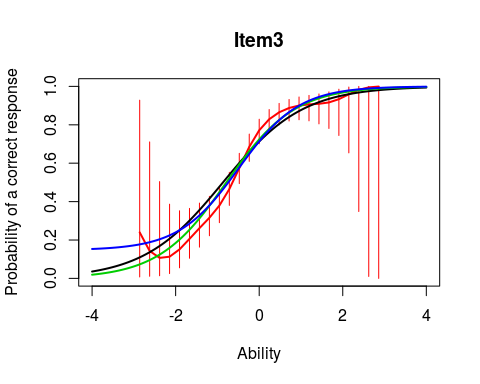Non-parametric curves are particularly valuable when applied to all response options, not just the correct response. Here is a plot for one of the more problematic items in the example data set. The irtoys function to produce such plots is now called tgf (previously known as tgp), and it needs to know the keys to the right answers:

keys = c(2,3,1,1,4,1,2,1,2,3,3,4,3,4,2,2,4,3)
tgf(choices=Unscored, key=keys, item=1, co=NA, label=TRUE, main="Item 1")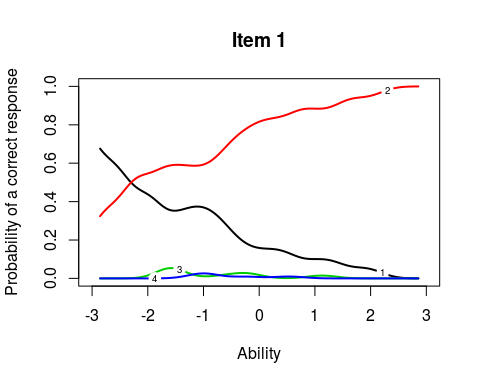The problem with Item 1 is that two of the distractors are so weak that practically no examinees chose them. For the less able examinees who might be inclined to guess, this reduces the problem to tossing a coin, and the logical value of the asymptote is 0.5 (BILOG-MG estimated it at 0.20776, so using a prior might be biasing down this time).

The qrs function starts with the data we actually observe, the sum scores, and translates them first to ranks and then to Normal deviates, in order to make them compatible with the metric of the latent variable. One might try the opposite: stretch and shrink the trace lines to bring them to the metric of the observed data, the sum scores. A similar idea has been proposed in the past by (Yen 1984). A new irtoys function, scoreMetric, tries to invert the test response function (TRF), i.e. select the values of $$\theta$$ at which the predicted test score comes closest to a given observed sum score:

p1 = scoreMetric(b1)
p2 = scoreMetric(b2)
p3 = scoreMetric(b3)

plot(0:ncol(Scored),  p1$f[,3], col=1, lwd=2, type="l", main="Item 3", xlab="Sum score", ylab="Probability") lines(0:ncol(Scored), p2$f[,3], co=3, lw=2)
lines(0:ncol(Scored), p3$f[,3], co=4, lw=2) plot(0:ncol(Scored), p1$f[,1], col=1, lwd=2, type="l", main="Item 1",
xlab="Sum score", ylab="Probability")
lines(0:ncol(Scored), p2$f[,1], co=3, lw=2) lines(0:ncol(Scored), p3$f[,1], co=4, lw=2)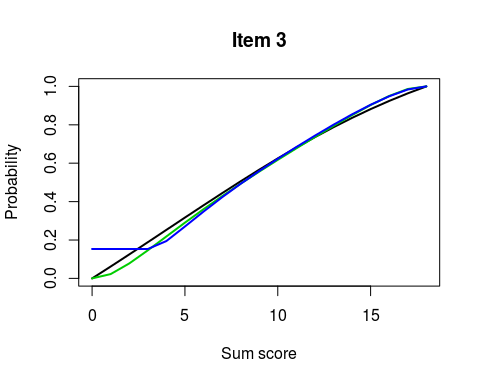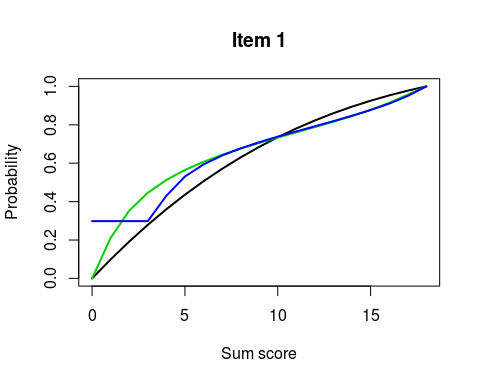The 1PL/Rasch model is shown in black, the 2PL model in green, and the 3PL model in blue. Note that these are not real item-total regressions but crude approximations, as computing the “real” expected probabilities at each sum score in not at all trivial except for the Rasch model. Then, an item-total regression must always start at (0,0) and end at (MaxTotalScore,1). This has been forced on most graphs with the exception of the 3PL model, which has some trouble in predicting a zero score anyway.

This brings us to the last and arguably most valuable addition to irtoys, function interactionModel, which fits Haberman’s interaction model (Haberman 2007) and the Rasch model by conditional maximum likelihood (CML).

The interaction model (IM) must be one of the best kept secrets in IRT. It is a heavily parameterized model that allows for conditional dependence among items and can be used routinely as an approximation to the data. In both respects, it differs from the Rasch model (RM), which assumes that items are conditionally independent given the sum score and has a reputation of hardly ever fitting the data.

The IM reproduces faithfully the two aspects of the test that really matter to the practitioner: the proportions correct and the item-total correlations. In other words, it captures everything interesting in the data and leaves out random noise. For practical purposes, comparing the Rasch model to the interaction model is like comparing the model to the data. And, visual comparison is easy because the interaction model preserves the conditioning properties of the RM – among other things, this means that it can be easily added to the plot:

rim(Scored, items=c(3,1), ncol=1, shade=0, show=TRUE)
## NULL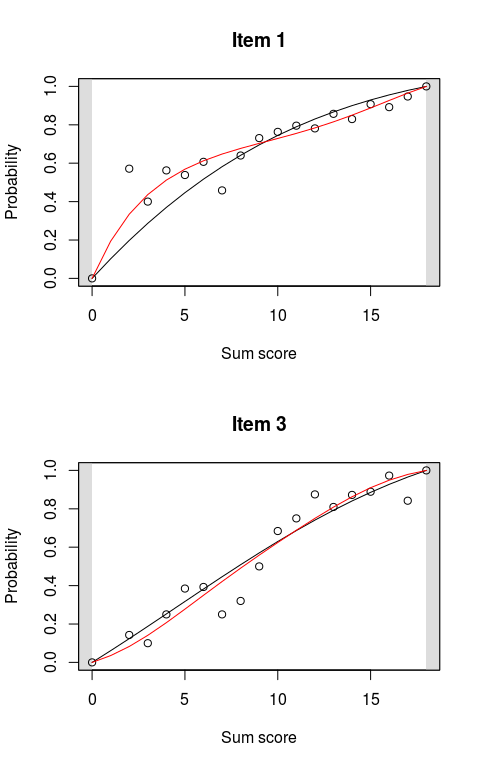Because the data points do not tell us much that the IM curves haven’t, we can omit them:

rim(Scored, items=c(3,1), ncol=1)
## NULL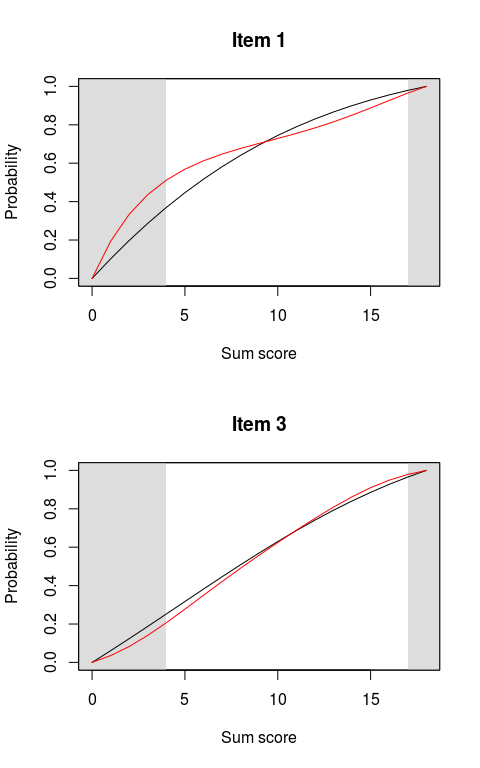Keeping the shade parameter at its default value of 10 (percent) shades the 10% most extreme and least frequently observed sum scores. On the left, just observing the item-total regression where the data really starts, in this case about a sum score of 4, arguably tells us more about guessing than that third parameter.

The IM is related to the 2PL model – in fact, the 2PL is a first-order approximation of the IM. Without a bit of experience, this may not be evident. The item-total regression must always start at (0,0) – if the total score is 0, then the item score must be 0 – and it always ends at (MaxTotalScore,1). To accommodate a particularly low discrimination, the line is forced to change shape, and then it looks like a logistic curve mirrored over the identity line. Such regressions have a negative interaction parameter, while the 2PL discrimination parameter will be low. To this writer, it usually means bad item quality – in fact, careful analysis of the distractors with function tgf will reveal problems.

When judging the “performance” of our items, it makes sense, then, to replace this plot:

flat = 1 + (b2\$est[,1] < .8)
plot(irf(b2), items=steep, label=TRUE, main="IRF for the 2PL model", co=flat, lw=1)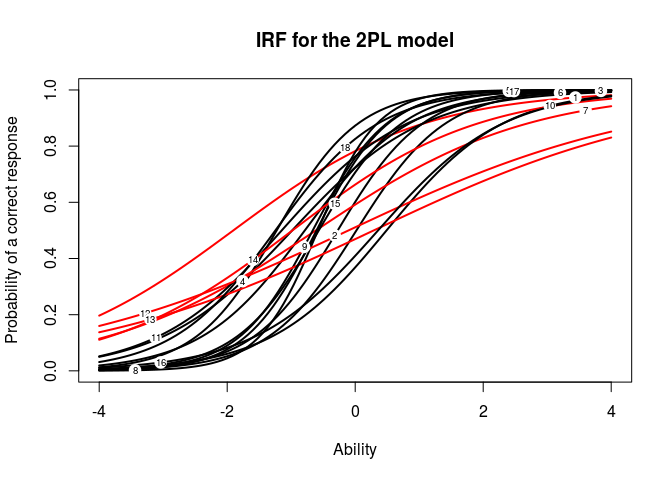with that:

plot(interactionModel(Scored), highlight=-.03, label=TRUE)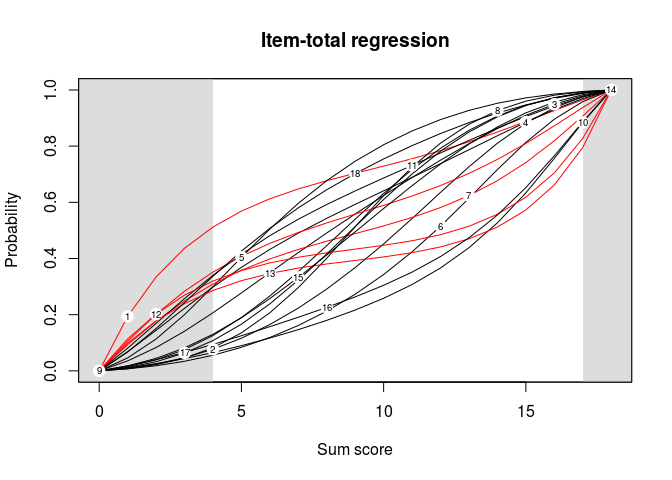In the first case, items having a discrimination less than 0.8 are highlighted, while in the second case items with an interaction parameter less than -.03 are highlighted. The advantages of the second graph over the first one should be clear from the above said.

1. KernSmoothIRT depends on package rgl. Installing the latter on Linux can be a small challenge as it depends on the Linux libraries, libglu1-mesa-dev, freeglut3-dev, mesa-common-dev, and possibly bwidget. This is meant as information and help for Linux users, not as a critique.